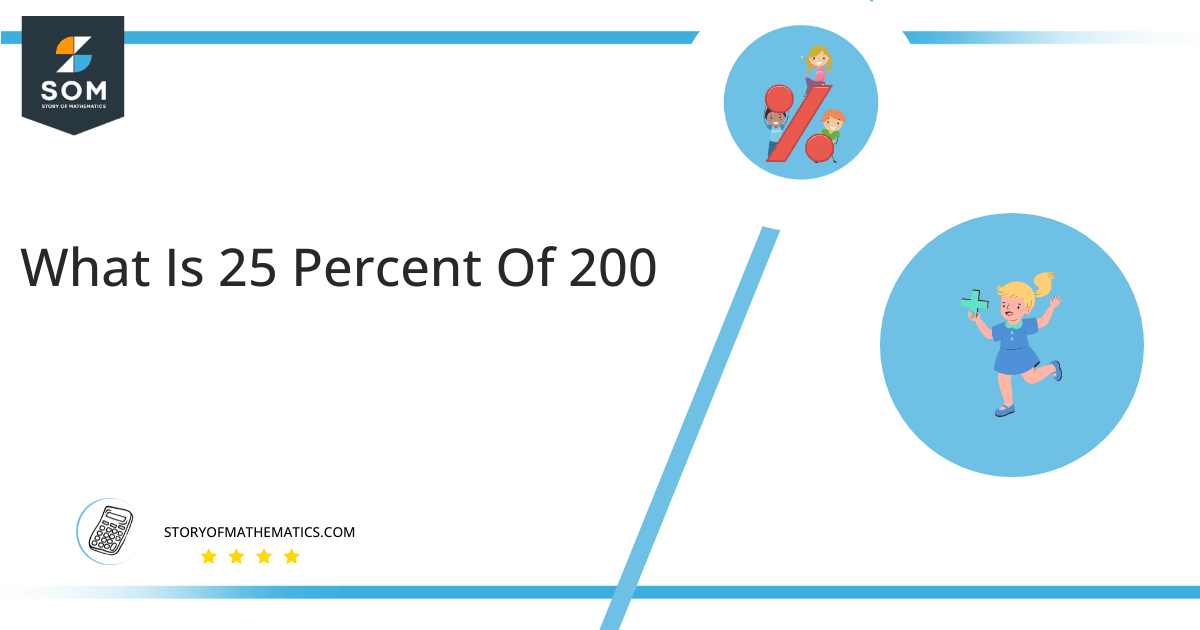# What Is 25 percent of 200 + Solution with Free StepsThe outcome of 25% of 200 is the number 50. This solution may be obtained by multiplying the component 0.25 by 200.

The twenty five percent of two hundred is very useful calculation for daily life. For example, suppose you were to buy a product on an online platform worth 200 US dollars. All of a sudden there is an end of season sale on the product worth 25% on the listed price. Since you know that 25 percent of 200 is equal to 50, you can clearly say that you will get a discount of 50 US dollars on the product. In short, you will have to pay only 150 US dollars instead of 200 US dollars.

In this article, the method for calculating a percentage of a given number is described. We’ll explain the procedure for figuring out what percentage of 200 is equal to 25%.

## What Is 25 percent of 200?

The result of 25 percent of 200 is 50. This result may be obtained by multiplying the component 0.25 by 200.

The solution to the question “25% of 200” may be found by multiplying 200 by a fraction of 25/100 and then reducing the result to a fractional number.

## How To Calculate 25 percent of 200?

The following easy mathematical procedures can help you find 25% of 200.### Step 1

Writing 25 percent of 200 in mathematical form:

25 percent of 200 = 25% x 200

### Step 2

Substitute the % symbol with the fraction 1/100:

25 percent of 200 = ( 25 x 1/100 ) x 200

### Step 3

Rearranging the above equation gives:

25 percent of 200 = ( 25 x 200 ) / 100

### Step 4

Multiplying 25 with 200:

25 percent of 200 = ( 5000 ) / 100

### Step 5

Dividing 5000 by 100:

25 percent of 200 = 50

Therefore, the 25 percent of 200 is equivalent to 50

We can visualize that 25 percent of 200 is equal to 50 by using the following pie chart.Figure 1: Pie Chart for 25 percent of 200

The orange portion of the preceding figure, which equals 50, represents 25 percent of 200. The figure’s overall area corresponds to the value of 200 in its entirety. The remaining 150, or 75% of the total 200, are represented by the green region.

The percentage is a technique in which physical quantities are converted on a scale of zero to a hundred for comparison.

All the Mathematical drawings/images are created using GeoGebra.